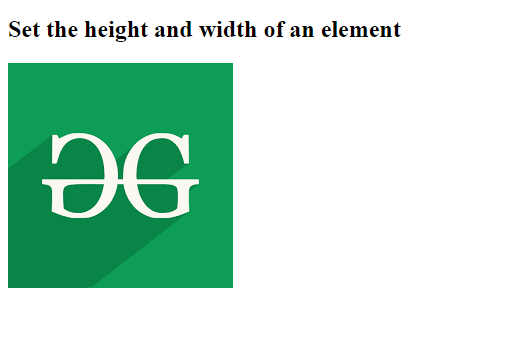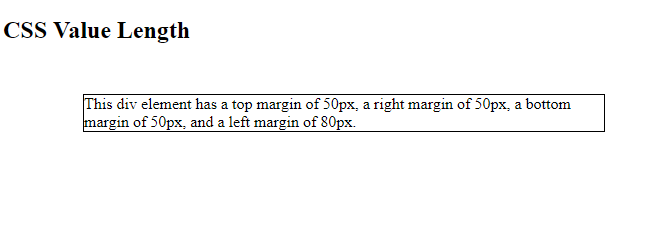Open in App
Not now

# CSS | Length Data Type

• Last Updated : 13 Jul, 2022

Length is the measurement of distance followed by a length unit. There is no space between the number and unit. It can be positive or negative. The length unit is optional after 0. There are two types of length units which are absolute and relative. Lengths are used in many CSS properties, such as padding, border-width, font-size, text-shadow, width, height, and margins.

Syntax:

`<length>`

It takes number followed by a unit of length as a parameter.

Note: No space should be given between the number and its unit.

Example 1: The following example demonstrates the use of length as value using CSS.

## html

 ```<``html``>` `<``head``>``    ``<``style``>``        ``div {``            ``height: 200px;``            ``width: 150px;``        ``}``    ``` `    ``<``title``>``        ``CSS | Length Data Type``    ````` `<``body``>``    ``<``h2``>``        ``Set the height and``        ``width of an element``    ``` `    ``<``div``>``        ``<``img` `src``=``"https://media.geeksforgeeks.org/wp-content/cdn-uploads/20190710102234/download3.png"``        ``alt``=``"GFG"``>``    ````` ``

Output:Example 2: The following example demonstrates the length as a value for margins of a div element.

## html

 ```<``html``>` `<``head``>``    ``<``style``>``        ``div {``            ``border: 1px solid black;``            ``margin-top: 50px;``            ``margin-bottom: 50px;``            ``margin-right: 50px;``            ``margin-left: 80px;``            ``background-color: lightblue;``        ``}``    ````` `<``body``>` `    ``<``h2``>CSS Value | Length` `    ``<``div``>``        ``This div element has a top``        ``margin of 50px, a right``        ``margin of 50px, a bottom``        ``margin of 50px, and a left``        ``margin of 80px.``    ````` ``

Output:Supported Browsers:

• Google Chrome 1 and above
• Edge 12 and above
• Internet Explorer 3 and above
• Firefox 1 and above
• Opera 3.5 and above
• Safari 1 and above

My Personal Notes arrow_drop_up